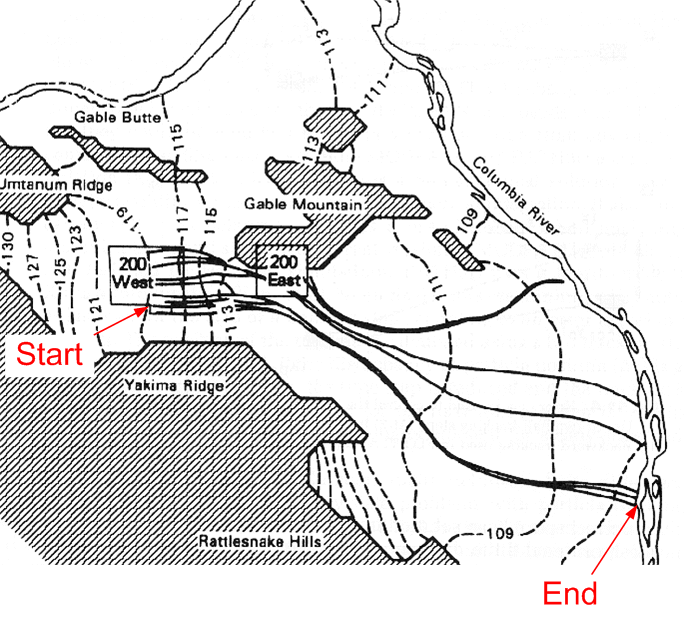# Travel Time Problem

Solution:

head change = dh ~ 119-106 = 13 m --> 13m*3.28ft/m = 42.6 ft

distance = dl = 20*5280 = 105600 ft

v = ki/ne = k(dh/dl)/ne = 1.5*(42.6/105600)/0.3 = 0.002 ft/d

v = x/t --> t = x/v

t = 105600/0.002 = 52.8 M days = 144,660 yrs